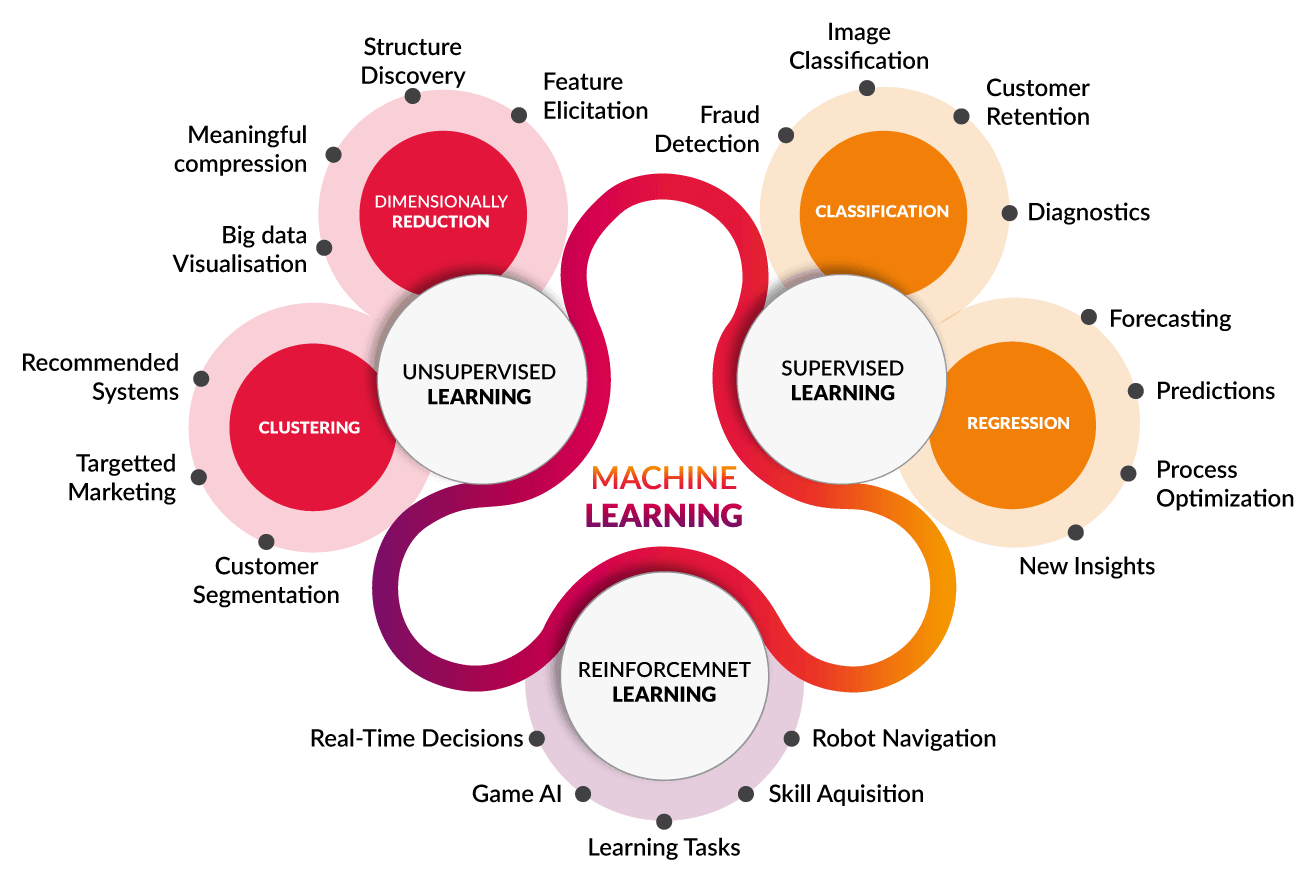July 12, 2019 - 12:06 pm

Introduction to Machine Learning

This is the era of Machine Learning (ML) as increasing the power of computation in computer technologies, and there is a lot of data to manage for each sector in the world. We have lots of data (symptoms, cures) for diseases, economic data (share market, trading) and there is also confidential data that could be used to decide on a business, agriculture, or even in a presidential decision for a country. These are the enormous use of ML, except that there are various small sectors where machine learning is performing well for doing human tasks.

Machine Learning is a significant branch of Artificial Intelligence (AI). The idea of machine learning is to generate a decision from several earlier examples for a new instance of the same kind of problem or cluster them in several groups.

Machine Learning is divided into three substantial categories, such as –

1. Supervised Learning
2. Unsupervised Learning
3. Reinforcement Learning

Let’s discuss about these three categories.

1. Supervised Learning

In this category of machine learning, we want to train the model with some features, and there is class value/values for each instance. Here,

1. Features are whatever things we need to express or describe the event/object; it could be size, time, length etc.
2. Class value is the thing what we want to predict about the object, like type etc.
3. Description of each event/object is an instance.

There are various models/algorithms to solve this kind of problem. However one model is better than other models for different contexts. So here are several machine learning models –

• Naive Bayes
• KNN
• Decision Tree
• Support Vector Machine
• Linear Regression
• Logistic Regression
• Deep Learning models – Most popular

We will discuss about deep learning in upcoming blog post in elaborately.

2. Unsupervised Learning

Unsupervised learning is the method to group or categories samples. Unlike machine learning, it does not have class values for the instances. Therefore it is mostly used to find previously unknown patterns. There is a couple of algorithms for this category-

• K-means
• DBSCAN
• OPTICS algorithm
• Mixture models

3. Reinforcement Learning

Reinforcement learning is the way of a train a model without human interaction. As a result the model learns interactively from the environment. So, when the model gets an event or object, it tries to predict the result that we want. Therefore if the result is correct, the model receives a reward, and if the result is wrong, the model gets punishment. In that way, the model knows what should do next time.

Tools for Machine Learning

There is a lot of tools for developing machine learning with different features and advantages; here, I am sharing the most popular tools. Such as-

• Frameworks for Machine Learning: sci-kit learn, PyTorch, TensorFlow, Keras
• Helper Frameworks: pandas, numpy, matplotlib, seaborn, OpenCV
• Programming Languages: Python, R, C++

Keep eyes for my next blog- Augnitive Blogs

••